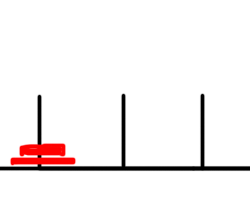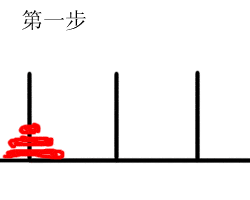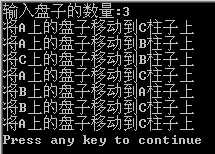# 汉诺塔问题1. hanota(n-1,A,C,B);
2. hanota(1,A,B,C);
3. hanota(n-1,B,A,C);

```#include<stdio.h>
void hanota(int n,char x,char y,char z){
if(1 == n)
printf("将%c上的盘子移动到%c柱子上\n",x,z);
else{
hanota(n-1,x,z,y);  //第一步
printf("将%c上的盘子移动到%c柱子上\n",x,z);  //第二步
hanota(n-1,y,x,z);  //第三步
}
}
int main(){
int n;
printf("输入盘子的数量:");
scanf("%d",&n);
hanota(n,'A','B','C');
return 0;
}
```# 赞赏# 评论@草没味的梨：盘子个数不同，移动的步骤当然不同呀